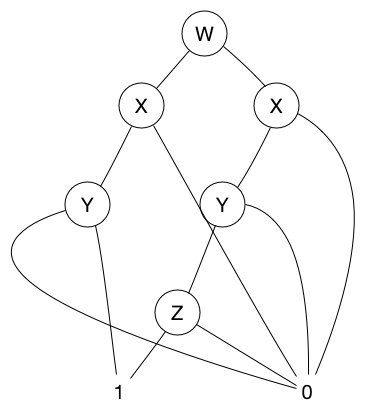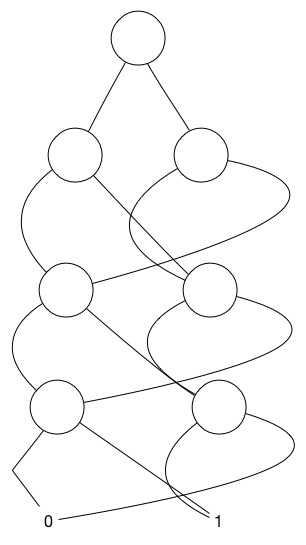• Introduction
• 1. 1.Introduction
• 2. 2.A Simple Syntax-Directed Translator
• 3. 3.Lexical Analysis
• 4. 4.Syntax Analysis
• 5. 5.Syntax-Directed Translation
• 6. 6.Intermediate-Code Generation
• 7. 7.Run-Time Environments
• 8. 8.Code Generation
• 9. 12.InterProcedural Analysis
• Published with GitBook

# Exercises for Section 12.7

### 12.7.1

Using the encoding of symbols in Example 12.28, develop a BDD that represents the relation consisting of the tuples (b,b), (c,a), and (b,a). You may order the boolean variables in whatever way gives you the most succinct BDD.### 12.7.2

As a function of n, how many nodes are there in the most succinct BDD that represents the exclusive-or function on n variables. That is, the functions is true if an odd number of the n variables are true and false if an even number are true.

For each variable, we put it on one layer. Their is only two possible arrangement left 0 or left 1. So for each variable, we need at most two nodes for it. And we need only one node for the first variable. So the answer is:
2n-1
For example, when n is 4:### 12.7.3

Modify Algorithm 12.29 so it produces the intersection (logical AND) of two BDD's.

a. BASIS: Zero variables. The BDD's must both be leaves, labeled either 0 or 1. The output is the leaf labeled 1 if `both` input are 1, or the leaf labeled 0 if `either` is 0.
b. INDEUCTION 2. ...The first of these BDD's represents the function that is true for all truth assignments that have y1 = 0 and that make `both` of the give BDD's true.